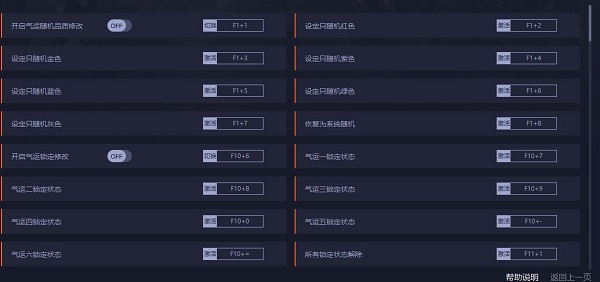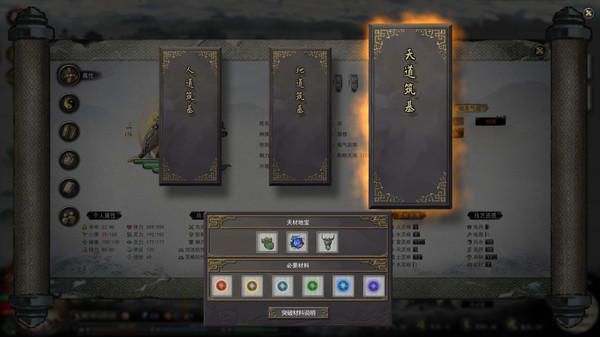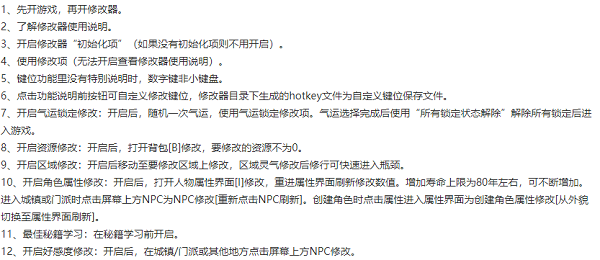• # 鬼谷八荒气运修改器

• 类型：游戏辅助 大小：23MB
• 更新: 2021-02-22 系统：
• 详情
• 相关
• 评论### 使用说明

F1+1 - 开启气运随机品质修改
F1+2 - 设定只随机红色
F1+3 - 设定只随机金色
F1+4 - 设定只随机紫色
F1+5 - 设定只随机蓝色
F1+6 - 设定只随机绿色
F1+7 - 设定只随机灰色
F1+8 - 恢复为系统随机
F10+6 - 开启气运锁定修改
F10+7 - 气运一锁定状态
F10+8 - 气运二锁定状态
F10+9 - 气运三锁定状态
F10+0 - 气运四锁定状态
F10+- - 气运五锁定状态
F10+= - 气运六锁定状态
F11+1 - 所有锁定状态解除### 注意事项### 键位说明

F1+9 - 开启战斗修改
F1+0 - 无限灵力
F1+- - 无限念力
F1+= - 生命回复
F2+6 - 灵力回复
F2+7 - 念力回复
F2+8 - 生命不减
F2+9 - 一击必杀
F2+0 - 开启资源修改
F2+- - 灵石+5000
F2+= - 最大灵石
F3+1 - 城主令+50
F3+2 - 城主令最大
F3+3 - 宗门贡献+5000
F3+4 - 宗门贡献最大
F3+5 - 开启区域修改
F3+6 - 灵气+1000
F3+7 - 最大灵气
F3+8 - 风水+1000
F3+9 - 最大风水
F3+0 - 最大药材
F3+- - 最大金石
F3+= - 超级速度
F4+1 - 开启角色属性修改
F4+2 - 增加寿命上限
F4+3 - 最大魅力
F4+4 - 最大正道
F4+5 - 最大魔道
F4+6 - 非正非魔
F4+7 - 声望+100
F4+8 - 最大声望
F4+9 - 道点+100
F4+0 - 道点最大
F4+- - 悟性+10
F4+= - 最大悟性
F5+1 - 当前健康回复
F5+2 - 健康上限最大
F5+3 - 当前体力回复
F5+4 - 体力上限最大
F5+5 - 当前灵力回复
F5+6 - 灵力上限最大
F5+7 - 当前念力回复
F5+8 - 念力上限最大
F5+9 - 幸运+10
F5+0 - 幸运最大
F5+- - 当前精力回复
F5+= - 精力上限最大
F6+1 - 当前心情回复
F6+2 - 心情上限最大
F6+3 - 攻击+100
F6+4 - 攻击最大
F6+5 - 防御+100
F6+6 - 防御最大
F6+7 - 脚力+100
F6+8 - 脚力最大
F6+9 - 会心+10
F6+0 - 会心最大
F6+- - 暴击倍数+10
F6+= - 暴击倍数最大
F7+1 - 护心+10
F7+2 - 最大护心
F7+3 - **倍数+10
F7+4 - **倍数最大
F7+5 - 移速+50
F7+6 - 移速设为350
F7+7 - 最大移速
F7+8 - 功法抗性+100
F7+9 - 功法抗性最大
F7+0 - 灵根抗性+100
F7+- - 灵根抗性最大
F7+= - 火灵根+10
F8+1 - 水灵根+10
F8+2 - 雷灵根+10
F8+3 - 风灵根+10
F8+4 - 土灵根+10
F8+5 - 木灵根+10
F8+6 - 所有灵根资质最大
F8+7 - 所有灵根资质设为10
F8+8 - 剑法+10
F8+9 - 枪法+10
F8+0 - 刀法+10
F8+- - 拳法+10
F8+= - 掌法+10
F9+1 - 指法+10
F9+2 - 所有功法资质最大
F9+3 - 所有功法资质设为10
F9+4 - 炼丹+10
F9+5 - 炼器+10
F9+6 - 风水+10
F9+7 - 画符+10
F9+8 - 药材+10
F9+9 - 矿材+10
F9+0 - 所有技艺资质最大
F9+- - 所有技艺资质设为10
F9+= - 时间停止
F10+1 - 游戏难度暂解锁
F10+2 - 最佳秘籍学习
F10+3 - 开启好感度修改
F10+4 - 好感度+60
F10+5 - 好感度最大
• 网游
• 单机
• 应用